### Calculate the spring constant for a linear spring given the spring potential energy and the extension displacement.

#### Example question:

A linear spring has a potential energy of 7.3 J when it is stretched 0.29 m. What is the constant for this spring?

#### Given:

spring potential energy: Us = 7.3 J

displacement: x = 0.29 m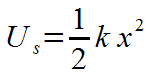Starting formula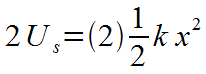Algebra: multiply each side by 2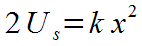Algebra: 2's cancel on right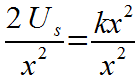Algebra: divide each side by x^2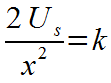Algebra: x^2's cancel on right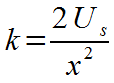Algebra: switch left and right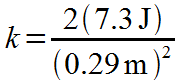Plug in numbers with units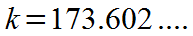Multiply: (2)(7.3)/0.29^2 = 173.602....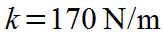Final result with significant figures and unit